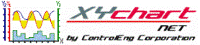XY Chart NET 3 Control Reference
AbsMinXValue Property

Use this property to return the absolute minimum x-value of a specific x-axis.

Syntax
 [Visual Basic] ReadOnly Property AbsMinXValue(XAxisNumber As Integer) As Double [C#] double XYChartNETCtl.get_AbsMinXValue(int XAxisNumber) [C++] property double AbsMinXValue[int]

Parameters
XAxisNumber
The x-axis index to obtain the absolute minimum value of.  Valid values are 0..(NumXScales - 1).
Remarks

Absolute minimum is the lowest value that is displayed on the graph for the specified x-axis. The value returned is of type Double.This property is read-only and should only be called after a Refresh call has been made.
Example
```' XYChartNETCtl1 is the name of the XY Chart NET control instance placed on the form.

Dim tmpMin As Double
Dim tmpMax As Double

tmpMin = XYChartNETCtl1.AbsMinXValue(1)  'Get the absolute min value for x-axis 1
tmpMax = XYChartNETCtl1.AbsMaxXValue(1)  'Get the absolute max value for x-axis 1
```
```// XYChartNETCtl1 is the name of the XY Chart NET control instance placed on the form.

double tmpMin;
double tmpMax;

tmpMin = XYChartNETCtl1.get_AbsMinXValue(1);  //Get the absolute min value for x-axis 1
tmpMax = XYChartNETCtl1.get_AbsMaxXValue(1);  //Get the absolute max value for x-axis 1
```
```// XYChartNETCtl1 is the name of the XY Chart NET control instance placed on the form.

double tmpMin;
double tmpMax;

tmpMin = XYChartNETCtl1->AbsMinXValue;  //Get the absolute min value for x-axis 0
tmpMax = XYChartNETCtl1->AbsMaxXValue;  //Get the absolute max value for x-axis 0
```